# Emitter follower help.

Gold Member
Hi all. So I have been trying to understand how a emitter follower (common collector) works. But I can't seem to grasp the physics behind it. I know it is used as a buffer, it has high input impedance and low output impedance. I don't know what properties cause the output voltage to follow the input voltage. Also if you have "The Art of Electronics," on page 67 where it says "Important points about followers," I can't understand why clipping occurs for the signal. For some reason this whole thing is kind of vague to me. Please help. Thank you.

## Answers and Replies

Gold Member
Hi
Without any Maths, you can say that current will pour into the base and, hence, through the transistor (current amplification factor of at least several tens) from C to E, until Vbe is around 0.7V. When that happens, Vout is 0.7V below Vin. If you vary Re, the current through the transistor will change to re-establish the 0.7V Vbe. There is so-called Voltage Feedback because any small change in Vout will cause a change in Ib and hence a large change in Ie - and this will maintain Vout to be what it was. This gives an effectively low output impedance - as the Volts are independent of the load resistance. If Vin is raised, the current that will be drawn through the base will not change appreciably because Ve 'chases Vb', remaining 0.7V below. So the current into the base of the Emitter follower will not change as Vin increases - which is what you would get with a very high resistance - hence the high input resistance.
Why does it 'limit'? If you try to take Vb too high then the transistor will saturate and no more current can pass through Re, so the volts cannot increase. The feedback mechanism runs out. Vbe need only increase by a miniscule amount beyond this and the forward biased be diode will pass loads of current. The input impedance will drop as Vb increases until it approaches that of a forward biased diode in series with Re.
There you are - no Maths and plenty of arm waving. Does it help?

Ratch
perplexabot,

But I can't seem to grasp the physics behind it.

The physics are the same as any BJT. Do you mean the topology of the common collector?

I know it is used as a buffer, it has high input impedance and low output impedance.

Its use won't help you understand how it works.

I don't know what properties cause the output voltage to follow the input voltage.

The property of any transistor in the active region. The emitter terminal voltage is around 0.7 volts different than the base terminal. So the emitter follows the base or vice versa voltage wise.

Also if you have "The Art of Electronics,

I don't, but clipping occurs whenever the input is overdriven or the operating point is chosen badly.

Ratch

Gold Member
It isn't clear at what level he's asking the question. I think the confusing phrase is "the physics behind it". Is it a real Physics question or a simple application question? I interpreted it as the latter. Very little 'Physics' is needed beyond V=IR, in a first stab at transistors. (Plus remembering the rules of how they behave).

Jony130
Emitter follower is a very simply circuit. The output voltage is always 0.6V lower the the input voltage.
See some examples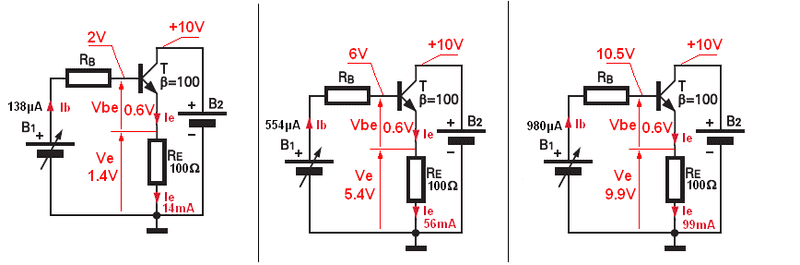Also notice that the base current is (β+1) smaller then emitter current (load current).
So our base current source B1 see our load ( Re resistor) not as 100Ω resistor. But B1 see (β+1)*Re load.
Here you have anther example.
Spouse we have a 1K resistor voltage divider supply from 10V battery.
Without any load connect to the output terminal of our voltage divider the output voltage is equal 5V. Now we connect a 100Ω load resistor across the output terminal.
And now our voltage divider output voltage drops from 5V to 0.83V. So we ruin our circuit.
To fix this issue we add a buffer (emitter follower) see the diagram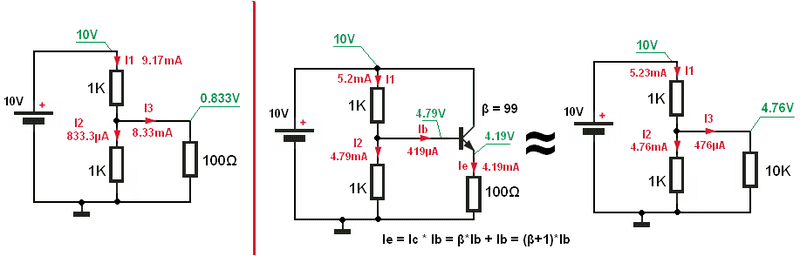Now I hope that you see why we say that emitter follower has a high input impedance and low output impedance. It's all thanks to BJT and his current gain.

#### Attachments

Gold Member
Thank you all for your great replies.

There is so-called Voltage Feedback because any small change in Vout will cause a change in Ib and hence a large change in Ie - and this will maintain Vout to be what it was. This gives an effectively low output impedance - as the Volts are independent of the load resistance.
Why will a change in Vout cause a change in Ib? Why would Vout change in the first place? Isn't Ib the one that is being "controlled" and so is subject to change?

I don't, but clipping occurs whenever the input is overdriven or the operating point is chosen badly.
May you please clarify this phrase? I don't understand what you mean by "overdriven or the operating point is chosen badly." Do you mean if Ib is low enough to cause Vbe to be lower than .7V then clipping shall occur?

See some examples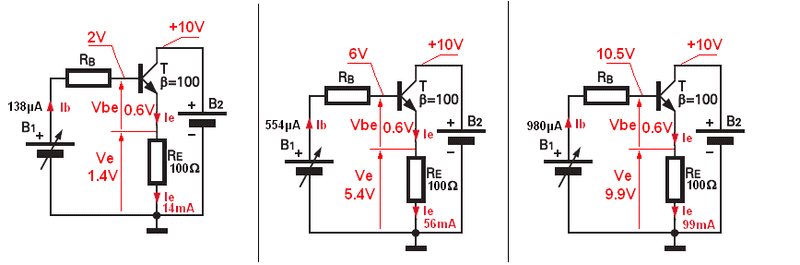For the first image, second figure, the current through Re, should it be 54mA?

Gold Member
I still don't understand page 67 of "The Art of Electronics" under "Important points about followers," number 1. If anyone can help that would be great.

Jony130
For the first image, second figure, the current through Re, should it be 54mA?
Yes, my mistake.

As for the clipping. The clipping can occur when transistor change his state eg. conduction to cut-off. See the example form The Art of Electronics
I slightly change the diagram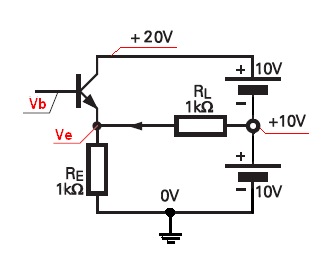And ask yourself one question. What voltage you need to provide to open BJT in this circuit. Also look at figure 2.9 in Art of Electronics.
Why will a change in Vout cause a change in Ib? Why would Vout change in the first place?
Vout can change for example if you change load resistance.

#### Attachments

Gold Member
Thank you for your help.

Yes, my mistake.

As for the clipping. The clipping can occur when transistor change his state eg. conduction to cut-off.
Cut-off happens at voltages less than .7v?

And ask yourself one question. What voltage you need to provide to open BJT in this circuit.
Is the answer .7V ?

Vout can change for example if you change load resistance.
That was what I was thinking, but I wasn't sure. Thanks.

On page 67 of "The Art of Electronics" it says:
The output can swing to within a transistor saturation voltage drop of Vcc, but it cannot go more negative than -5 volts. That is because on the extreme negative swing, the transistor can do no more than turn off, which it does at -4.4 volts input (-5V output).

I don't understand why clipping occurs at -5V (or -4.4V input) when cut-off happens at .7V. In other words shouldn't clipping occur at .7V?

Jony130
Thank you for your help.

Cut-off happens at voltages less than .7v?

Is the answer .7V ?

That was what I was thinking, but I wasn't sure. Thanks.

On page 67 of "The Art of Electronics" it says:
The output can swing to within a transistor saturation voltage drop of Vcc, but it cannot go more negative than -5 volts. That is because on the extreme negative swing, the transistor can do no more than turn off, which it does at -4.4 volts input (-5V output).

I don't understand why clipping occurs at -5V (or -4.4V input) when cut-off happens at .7V. In other words shouldn't clipping occur at .7V?
No 0.7V Is a Vbe voltage not the base voltage or load voltage.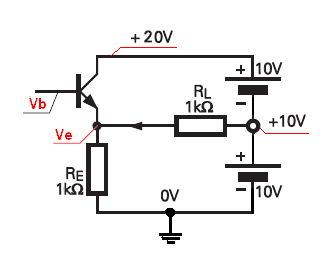In this imagine without BJT the Ve voltage is equal 5V so to open the BJT Vb voltage must be higher then 5.6V. So If input voltage is greater than 5.6V the BJT is active mode and the voltage across the load resistor is Vout = Vin - Vbe. But if Vb is smaller than the 5.6V the BJT is in cut-off stage. And the Ve voltage is constant 5V. Input voltage don't affect the VE voltage any more because BJT is cut-off.

Last edited:
Gold Member
No 0.7V Is a Vbe voltage not the base voltage or load voltage.In this imagine without BJT the Ve voltage is equal 5V so to open the BJT Vb voltage must be higher then 5.6V. So If input voltage is greater then 5.6V the BJT is active mode and the voltage across the load resistor is Vout = Vin - Vbe. But if Vb is smaller than the 5.6V the BJT is in cut-off stage. And the Ve voltage is constant 5V. Input voltage don't affect the VE voltage any more because BJT is cut-off.

Thank you so much that makes so much sense. Continuing on your example (assuming Ve is 5v when BJT is off), if Vin is 7v then Ve will be 6.3V, is that correct? Also does it saturate at 20V?

Last edited:
Jony130
Thank you so much that makes so much sense. Continuing on your example, if Vin is 7v then Ve will be 6.3V, is that correct? Also does it saturate at 20V?
Yes for Vb= 7V The output voltage Ve ≈ 6.3V
As for the saturation for Vb = 20V bjt is almost enter the saturation region.
To enter saturation Vb must be higher the Vcc.

Ok So now if we change the GND position as you have in Art of Electronics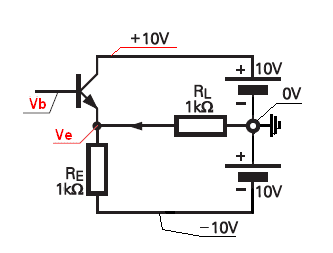I hope that now you see that without BJT we have VE = -5V. And now if you want to open the BJT the Vb voltage must we greater (lees negative) than -4.4V. If Vb is lower (more negative) than -4.4V the BJT is in cut-off mode.

#### Attachments

Last edited:
Gold Member
Yes for Vb= 7V The output voltage Ve ≈ 6.3V
As for the saturation for Vb = 20V bjt is almost enter the saturation region.
To enter saturation Vb must be higher the Vcc.

Ok So now if we change the GND position as you have in Art of ElectronicsI hope that now you see that without BJT we have VE = -5V. And now if you want to open the BJT the Vb voltage must we greater (lees negative) then -4.4V. If Vb is lower (more negative) the -4.4V the BJT is in cut-off mode.

Truly amazing explanation. I finally understand. Thank you so much.
Sorry for my many questions. With the BJT in cut-off mode, can it be treated as an open circuit (or infinite resistance)?

Last edited:
Jony130
With the BJT in cut-off mode, can it be treated as an open circuit (or infinite resistance)?
You can even delete BJT from the diagram.

Gold Member
You can even delete BJT from the diagram.
That simplifies a lot.

Once again thank you so much, you have been a great deal of help.

Gold Member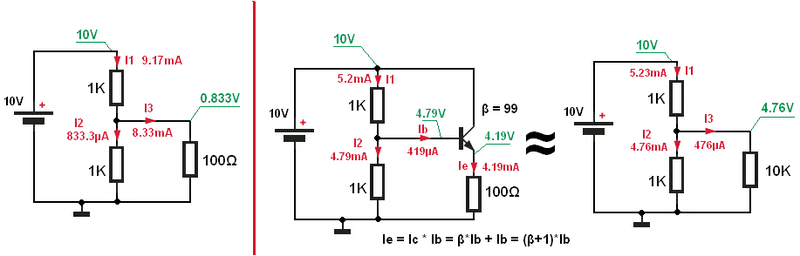How did you solve for Vb or Ib in the second circuit?

Jony130
I use a circuit theory and solve the circuit.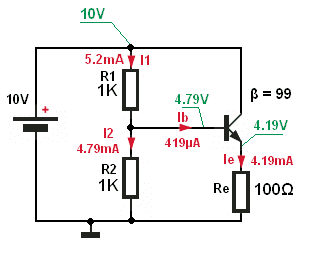From the II Kirchhoff's law we can write

Vcc = I1*R1 + I2*R2 (1)

I1 = Ib + I2 (2)

I2*R2 = Vbe + Ie*Re (3)

And Ib = Ie/(β+1) (4)

And we can solve this for Ib.

$\LARGE {I_b = \frac{R2Vcc - Vbe(R1+R2)}{(\beta +1)Re(R1+R2) + (R1R2)}}= 419.047619\mu A$ I assume β = 99 and Vbe = 0.6V

Knowing Ib we can easy solve for Ie. Ve and Vb

But there is also a simpler way to solve this circuit by using thevenin's theorem.
We can replace the voltage divider (this gray rectangle) with his Thevenin's equivalent circuit

Vth = Vcc * R2/(R1+R2)

Rth = R1||R2 = (R1*R2)/(R1+R2)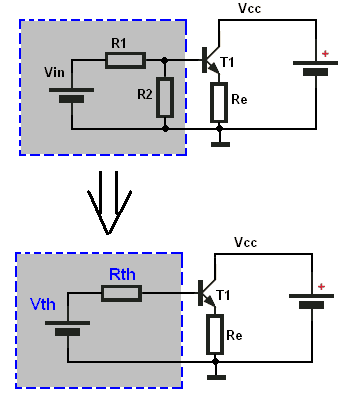And now we can solve for Ib

Vth - Ib*Rth - Vbe - Ie*Re = 0

And we also know that

Ie = Ib*(β +1)

so we end up with

Vth - Ib*Rth - Vbe - Ib*(β +1)*Re = 0

$\LARGE Ib = \frac{Vth-Vbe}{Rth+(\beta +1)*Re}$

And this is the end.

#### Attachments

Last edited:
•1 person
Gold Member
Most of what you wrote made sense but I do have 3 questions.

From the II Kirchhoff's law we can write

Vcc = I1*R1 + I2*R2 (1)

I1 = Ib + I2 (2)

I2*R2 = Vbe + Ie*Re (3)

And Ib = Ie/(β+1) (4)
How did you get equation (1)? Is Vcc = 10V, if Yes, then I think I get it.

But there is also a simpler way to solve this circuit by using thevenin's theorem.
We can replace the voltage divider (this gray rectangle) with his Thevenin's equivalent circuit

Vth = Vcc * R2/(R1+R2)

Rth = R1||R2 = (R1*R2)/(R1+R2)
I am familiar with thevenin's theorem, but I don't know how you got Vth = Vcc * R2/(R1+R2)? Once again if Vcc = 10V, then I think I understand.And now we can solve for Ib

Vth - Ib*Rth + Vbe + Ie*Re = 0
Isn't this supposed to be: Vth - Ib*Rth - Vbe - Ie*Re = 0 ?
Or are you assuming the emitter current going from ground to transistor?

I am sorry if I am asking for too much. You are helping me a lot.

Jony130
Vcc is a supply voltage Vcc = 10V in our example.

Isn't this supposed to be: Vth - Ib*Rth - Vbe - Ie*Re = 0 ?
Or are you assuming the emitter current going from ground to transistor?
No I made a typing error. Point for you.
I edit my post and correct the error.

Gold Member
Thank you.I have one last question for you (I hope). For the image above (top figure), did you just "copy/paste" Vin from collector to ground?

Jony130
I don't understand you question.
In our case Vin = Vcc = 10V. But sometimes Vin has a different value than supply voltage.

Gold MemberIn this figure you have only one battery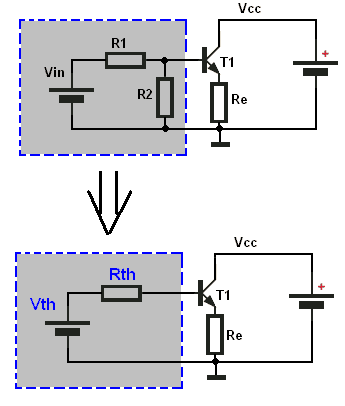In the top figure you have two batteries. How did you get the second one? Or are these two figures NOT equivelent?

Jony130
This two circuit are equivalent only when Vin = Vcc.
And yes I just just "copy/paste" Vin from collector to ground.
But also kept in mind that this second circuit with two batters is more universal for education purpose. Because Vin need not be equal to Vcc (supply voltage).

Gold Member
This two circuit are equivalent only when Vin = Vcc.
And yes I just just "copy/paste" Vin from collector to ground.
But also kept in mind that this second circuit with two batters is more universal for education purpose. Because Vin need not be equal to Vcc (supply voltage).

Thank you very much for your help.

Gold Member
Thank you all for your great replies.
Why will a change in Vout cause a change in Ib? Why would Vout change in the first place? Isn't Ib the one that is being "controlled" and so is subject to change?
This is basic approach to feedback theory.
An emitter follower is a circuit with Feedback. That accounts for the way that the output impedance is a lot lower than the value of the emitter load Re. If you want to analyse how feedback works (this is a general principle) you introduce an error signal and see the effect of the circuit on correcting this error. A reason that Vout could change could be that Re changes (or even that the current gain changes a bit). The feedback mechanism (involving the very high current gain of the transistor) corrects this by adjusting the Emitter current and bringing it back to (very near) what it was. When an output voltage is controlled to be more or less independent of the load, this is known as a voltage source or low impedance source. This basic approach may be unfamiliar to you but it is the way that we can see how circuits can be linearised, their frequency response flattened and their input and output impedances controlled.

You can use the methods described elsewhere in this thread to analyse what the circuit will do and they will give answers but you would need to do the sums, piecewise, for a range of values of Re, say. Approaching it as a feedback problem, you get the effective output impedance (etc.) irrespective of the value of Re. The same approach is used, of course, in amplifiers using Op Amps and simplifies many amplifier circuit problems significantly by omitting many of the less relevant circuit values.

Gold Member
This is basic approach to feedback theory.
An emitter follower is a circuit with Feedback. That accounts for the way that the output impedance is a lot lower than the value of the emitter load Re. If you want to analyse how feedback works (this is a general principle) you introduce an error signal and see the effect of the circuit on correcting this error. A reason that Vout could change could be that Re changes (or even that the current gain changes a bit). The feedback mechanism (involving the very high current gain of the transistor) corrects this by adjusting the Emitter current and bringing it back to (very near) what it was. When an output voltage is controlled to be more or less independent of the load, this is known as a voltage source or low impedance source. This basic approach may be unfamiliar to you but it is the way that we can see how circuits can be linearised, their frequency response flattened and their input and output impedances controlled.

You can use the methods described elsewhere in this thread to analyse what the circuit will do and they will give answers but you would need to do the sums, piecewise, for a range of values of Re, say. Approaching it as a feedback problem, you get the effective output impedance (etc.) irrespective of the value of Re. The same approach is used, of course, in amplifiers using Op Amps and simplifies many amplifier circuit problems significantly by omitting many of the less relevant circuit values.

Hello again. I have dealt with feedback before in op-amps, however the idea was hard for me to think about. I can understand the fact that feed back "corrects" changes of Vout so that it goes back to what it was but I don't know how this happens. That is why I am happy that you brought this subject up. When I dealt with op-amps, I treated them as black boxes and didn't think about how this can happen. Here is my reasoning of why feedback occurs in a transistor, please tell me if I am right/wrong or am missing something:

Vout changes due to noise, or a change in Re or some other reason and so Ie changes and since Ib = Ie + Ic, Ib will change as well, the change in Ib will change Vbe which in turn increases/decreases the Base-Emitter depletion region width causing more/less carries to pass through which changes Vout back to what it was.

What do you think?

Jony130
Vout changes due to noise, or a change in Re or some other reason and so Ie changes and since Ib = Ie + Ic, Ib will change as well, the change in Ib will change Vbe which in turn increases/decreases the Base-Emitter depletion region width causing more/less carries to pass through which changes Vout back to what it was.
Your description is quite correct except Ib equation.
In BJT Ie = Ib + Ic and Ic = Ib*β
So we have
Ie = Ib + Ib*β = Ib *(β+1)
And we solve for Ib
Ib = Ie/(β+1)

Also you can look at this circuit that Vbe is a cause of a Ib changes.
If Vout changes and Vin remains unchanged the Vbe also change because
Vbe = Vin - Vout. And change in Vbe Ib also change.

And in deep op amp analysis you can find here
https://www.physicsforums.com/showpost.php?p=3866238&postcount=3
I recommend you to read this

Gold Member
Thank you for the reply and the informative link.

In the link you say:

Now suppose the output voltage tried to drift in a positive direction. Can you see that this positive change would be felt through R2 and would cause the inverting pin (-) of the op amp to become slightly positive Since essentially no current flows in or out of the op amp input and the (+) input of the op amp is at ground potential. This causes VD to be greater than 0 with the (-) terminal being the most positive.

Shouldn't VD be LESS than 0? Because VD = V"+" - V"-" where V"+" = 0 and V"-" is positive so VD = 0 - (POSITIVE #) = a negative VD < 0

Last edited:
Jony130
Shouldn't VD be LESS than 0? Because VD = V"+" - V"-" where V"+" = 0 and V"-" is positive so VD = 0 - (POSITIVE #) = a negative VD < 0
Well you right VD is negative, but this description corresponds to this drawing
https://www.physicsforums.com/attachment.php?attachmentid=46245&stc=1&d=1334484384
But as you can see both of these methods gives the correct (the same) answer.
Negative VD forces the output voltage to decrease its value.

Gold Member
Another question about a BJT, how does a change in Ib produce a change in Vbe (on a microscopic level)?

Also as you increase the forward biased voltage across the Base-Emitter depletion region the depletion width decreases, right? Well, can you increases the voltage so much that the depletion width is effectively gone (is this even a valid question?) ?

Jony130
Another question about a BJT, how does a change in Ib produce a change in Vbe (on a microscopic level)?

Also as you increase the forward biased voltage across the Base-Emitter depletion region the depletion width decreases, right? Well, can you increases the voltage so much that the depletion width is effectively gone (is this even a valid question?) ?
I don't know, you must ask some physics. I'm not interested in this subject. And it is irrelevant from the application point of view.

Gold Member
Thank you for all your help.

Anyone?

Gold Member
Another question about a BJT, how does a change in Ib produce a change in Vbe (on a microscopic level)?
A change in Ib causes a change of carriers in the Base region, this causes an attraction/repulsion of the carriers in the Emmiter region, which causes the Base-Emitter depletion region to change width, this width correlates to a change in Vbe, but why?

Also as you increase the forward biased voltage across the Base-Emitter depletion region the depletion width decreases, right? Well, can you increases the voltage so much that the depletion width is effectively gone (is this even a valid question?) ?
I still don't know the answer to this?

Ratch
perplexabot,

Another question about a BJT, how does a change in Ib produce a change in Vbe (on a microscopic level)?

A BJT is a voltage controlled current source. Whatever voltage you put on the base-emitter will determine the current of the collector. The voltage input/current output is exponential, so designers use a lot of resistance in the base to swamp out the exponential variation. That is why they use a current source (high resistance) in the base to drive the transistor. A lot of folks think that a BJT is controlled by the base current, but it is not. It is controlled by Vbe in an exponential way according to Schockley's diode equation. The base current is also controlled by Vbe in a exponential manner. So the base current is related to the collector current is a somewhat linear manner through Vbe, but it is Vbe that is controlling both. So the base current is an indicator of the collector current, but does not control it. The reason for the exponential relationship is that a BJT operates by diffusion, whereas a FET and a vacuum tube operates by electrostatic means.

Also as you increase the forward biased voltage across the Base-Emitter depletion region the depletion width decreases, right? Well, can you increases the voltage so much that the depletion width is effectively gone (is this even a valid question?) ?

The emitter-base junction is just like a diode in that if you put too much voltage across it, it will burn out. That will happen before you can close the depletion region.

Ratch

Gold Member
perplexabot,

A BJT is a voltage controlled current source. Whatever voltage you put on the base-emitter will determine the current of the collector.
I think I am lost, doesn't the voltage drop across base-emitter have to be .7? Just like a diode? So if you apply a voltage of 3V, .7V will drop across the Base-Emitter, but happens to the other 2.3V?

The emitter-base junction is just like a diode in that if you put too much voltage across it, it will burn out. That will happen before you can close the depletion region.
Ratch

By "burn out," does it dissipate heat until it literally burns itself out?

Last edited: